# Recursion Chapter 10 Chapter Contents What Is Recursion

• Slides: 29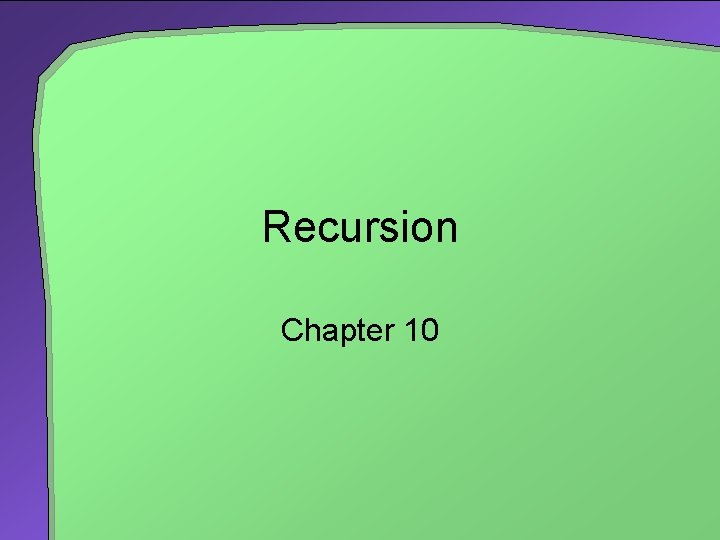Recursion Chapter 10Chapter Contents What Is Recursion? Tracing a Recursive Methods That Return a Value Recursively Processing an Array Recursively Processing a Linked Chain The Time Efficiency of Recursive Methods • Time Efficiency of count. Down • Time Efficiency of computing xn A Simple Solution to a Difficult Problem A Poor Solution to a Simple Problem Tail Recursion Mutual Recursion 2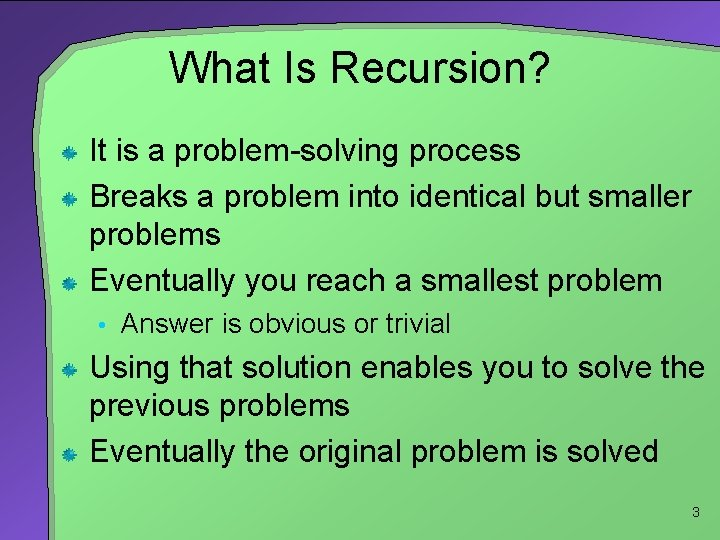What Is Recursion? It is a problem-solving process Breaks a problem into identical but smaller problems Eventually you reach a smallest problem • Answer is obvious or trivial Using that solution enables you to solve the previous problems Eventually the original problem is solved 3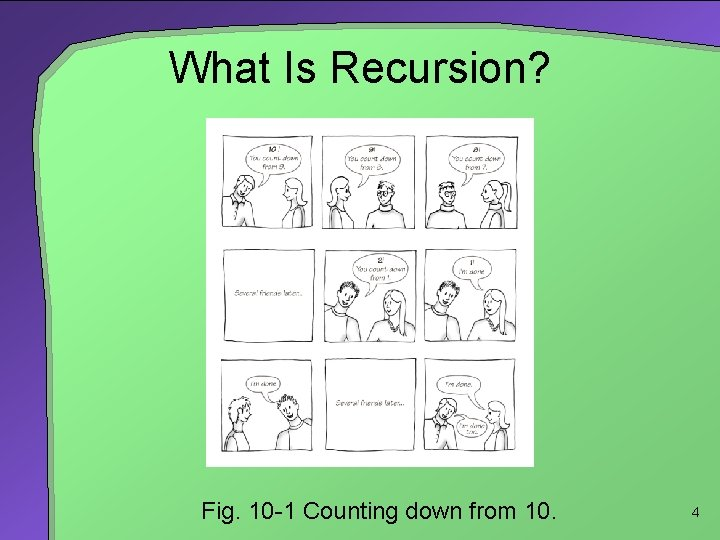What Is Recursion? Fig. 10 -1 Counting down from 10. 4What Is Recursion? A method that calls itself is a recursive method /** Task: Counts down from a given positive integer. * @param integer an integer > 0 */ public static void count. Down(int integer) { System. out. println(integer); if (integer > 1) count. Down(integer - 1); } // end count. Down The invocation is a • Recursive call • Recursive invocation 5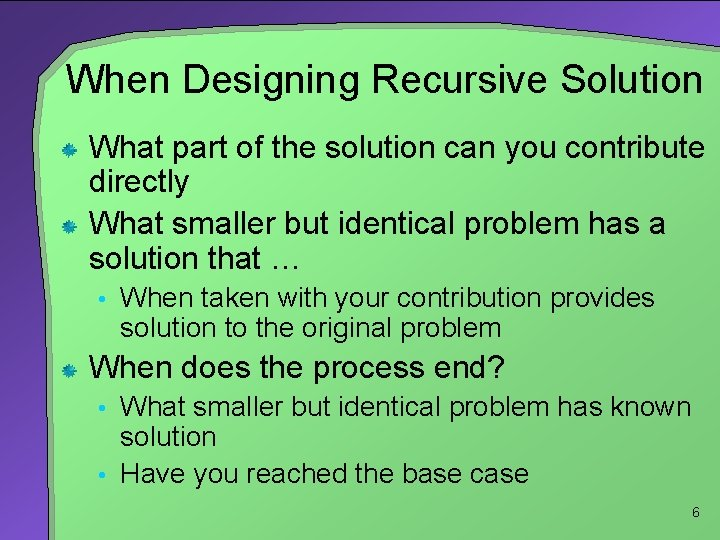When Designing Recursive Solution What part of the solution can you contribute directly What smaller but identical problem has a solution that … • When taken with your contribution provides solution to the original problem When does the process end? • What smaller but identical problem has known solution • Have you reached the base case 6When Designing Recursive Solution Method definition must provide parameter • Leads to different cases • Typically includes an if or a switch statement One or more of these cases should provide a non recursive solution • The base or stopping case One or more cases includes recursive invocation • Takes a step towards the base case 7Tracing a Recursive Method Given: public static void count. Down(int integer) { System. out. println(integer); if (integer > 1) count. Down(integer - 1); } // end count. Down Fig. 10 -2 The effect of method call count. Down(3) 8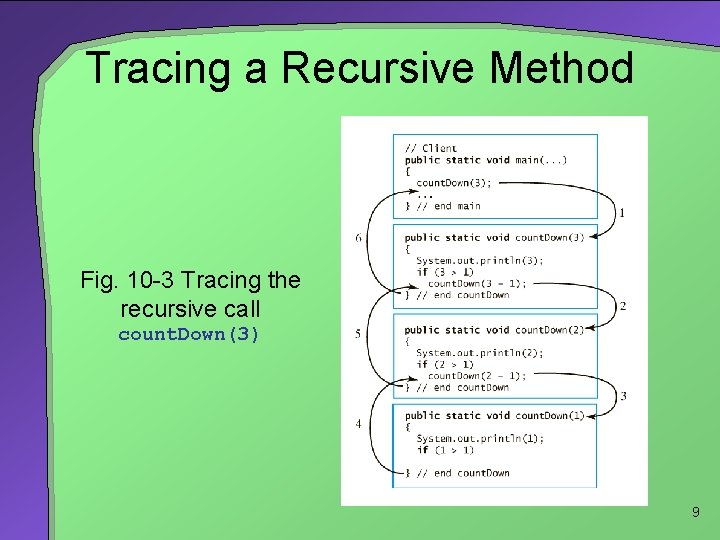Tracing a Recursive Method Fig. 10 -3 Tracing the recursive call count. Down(3) 9Tracing a Recursive Method Fig. 10 -4 The stack of activation records during the execution of a call to count. Down(3)… continued → 10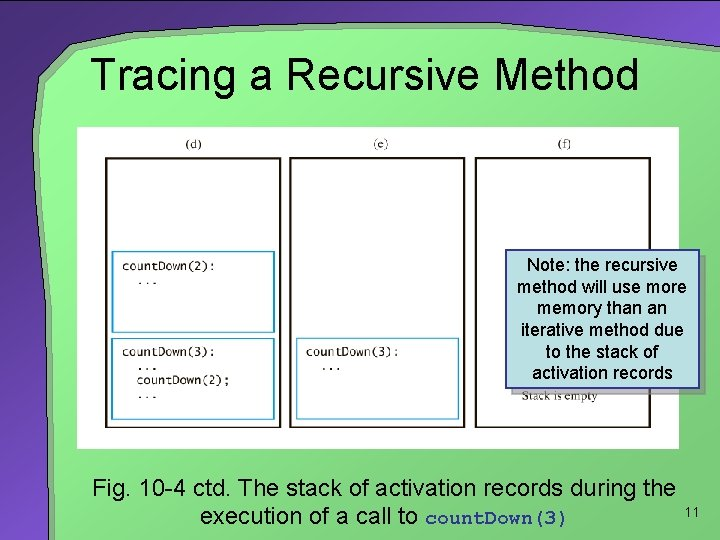Tracing a Recursive Method Note: the recursive method will use more memory than an iterative method due to the stack of activation records Fig. 10 -4 ctd. The stack of activation records during the execution of a call to count. Down(3) 11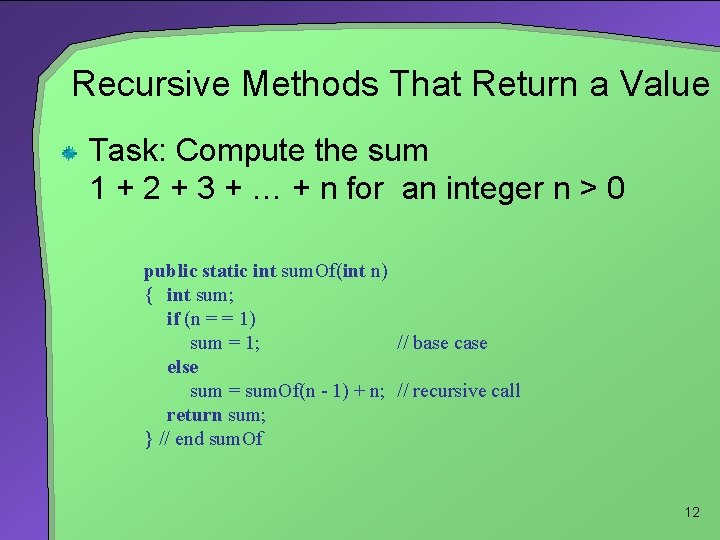Recursive Methods That Return a Value Task: Compute the sum 1 + 2 + 3 + … + n for an integer n > 0 public static int sum. Of(int n) { int sum; if (n = = 1) sum = 1; // base case else sum = sum. Of(n - 1) + n; // recursive call return sum; } // end sum. Of 12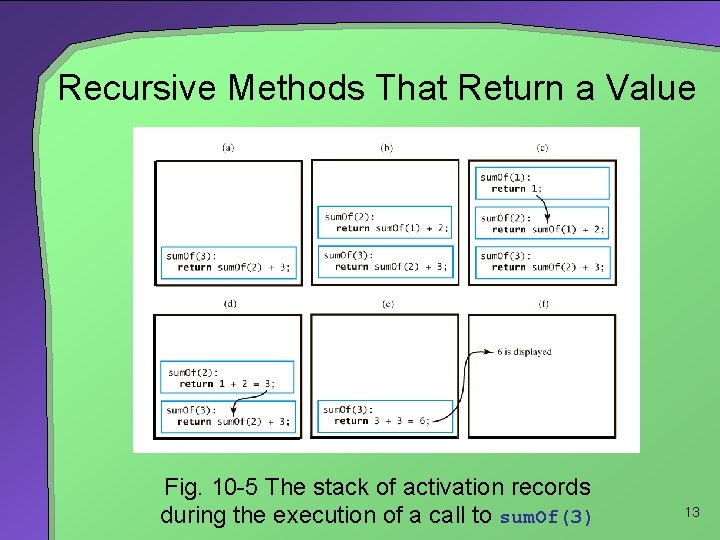Recursive Methods That Return a Value Fig. 10 -5 The stack of activation records during the execution of a call to sum. Of(3) 13Recursively Processing an Array When processing array recursively, divide it into two pieces • Last element one piece, rest of array another • First element one piece, rest of array another • Divide array into two halves A recursive method part of an implementation of an ADT is often private • Its necessary parameters make it unsuitable as an ADT operation 14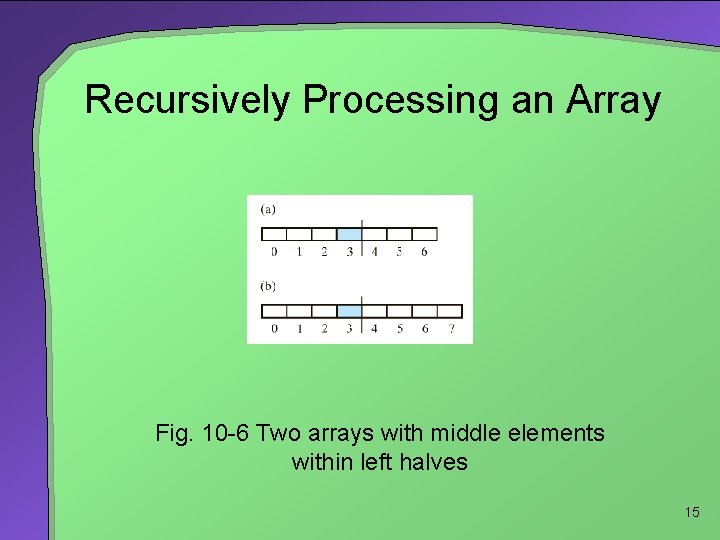Recursively Processing an Array Fig. 10 -6 Two arrays with middle elements within left halves 15Recursively Processing a Linked Chain To write a method that processes a chain of linked nodes recursively • Use a reference to the chain's first node as the method's parameter Then process the first node • Followed by the rest of the chain public void display() { display. Chain(first. Node); System. out. println(); } // end display private void display. Chain(Node node. One) { if (node. One != null) { System. out. print(node. One. data + " "); display. Chain(node. One. next); } } // end display. Chain 16Time Efficiency of Recursive Methods For the count. Down method public static void count. Down(int integer) { System. out. println(integer); if (integer > 1) count. Down(integer - 1); } // end count. Down • The efficiency is O(n) For computing xn recursively • The efficiency is O(log n) 17A Simple Solution to a Difficult Problem Fig. 10 -7 The initial configuration of the Towers of Hanoi for three disks 18A Simple Solution to a Difficult Problem Rules for the Towers of Hanoi game 1. Move one disk at a time. Each disk you move must be a topmost disk. 2. No disk may rest on top of a disk smaller than itself. 3. You can store disks on the second pole temporarily, as long as you observe the previous two rules. 19A Simple Solution to a Difficult Problem Fig. 10 -8 The sequence of moves for solving the Towers of Hanoi problem with three disks. Continued → 20A Simple Solution to a Difficult Problem Fig. 10 -8 (ctd) The sequence of moves for solving the Towers of Hanoi problem with three disks 21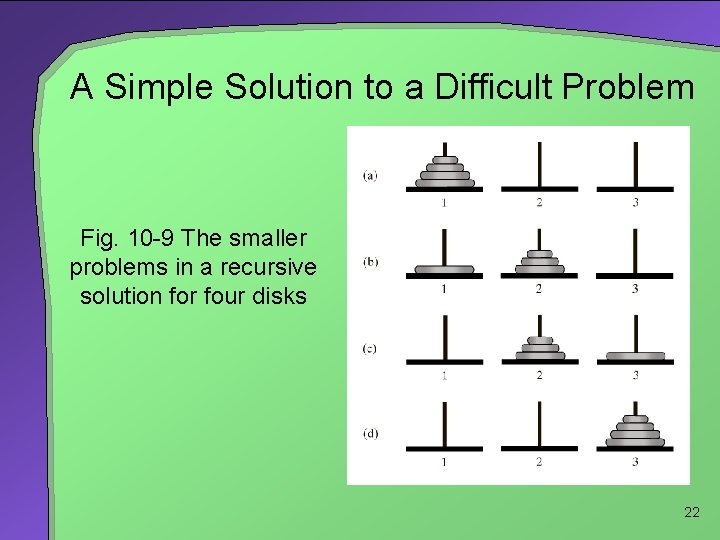A Simple Solution to a Difficult Problem Fig. 10 -9 The smaller problems in a recursive solution for four disks 22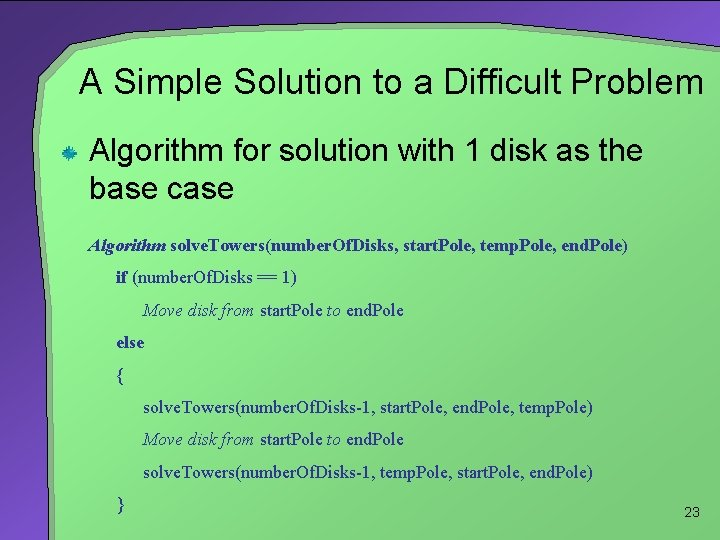A Simple Solution to a Difficult Problem Algorithm for solution with 1 disk as the base case Algorithm solve. Towers(number. Of. Disks, start. Pole, temp. Pole, end. Pole) if (number. Of. Disks == 1) Move disk from start. Pole to end. Pole else { solve. Towers(number. Of. Disks-1, start. Pole, end. Pole, temp. Pole) Move disk from start. Pole to end. Pole solve. Towers(number. Of. Disks-1, temp. Pole, start. Pole, end. Pole) } 23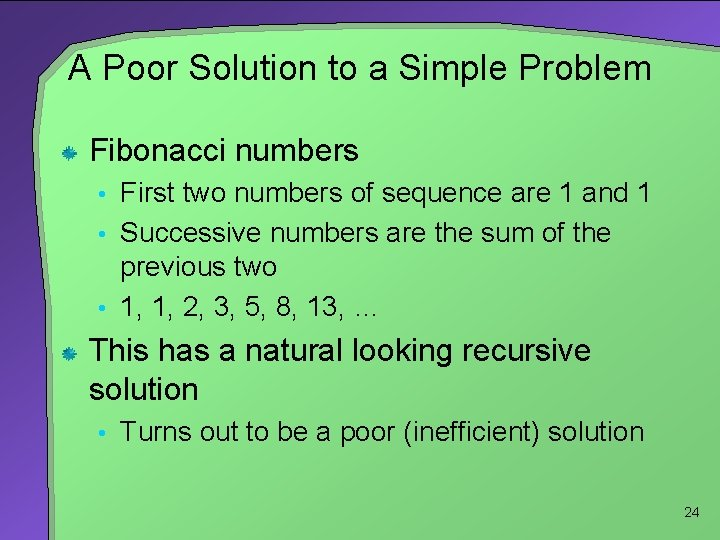A Poor Solution to a Simple Problem Fibonacci numbers • First two numbers of sequence are 1 and 1 • Successive numbers are the sum of the previous two • 1, 1, 2, 3, 5, 8, 13, … This has a natural looking recursive solution • Turns out to be a poor (inefficient) solution 24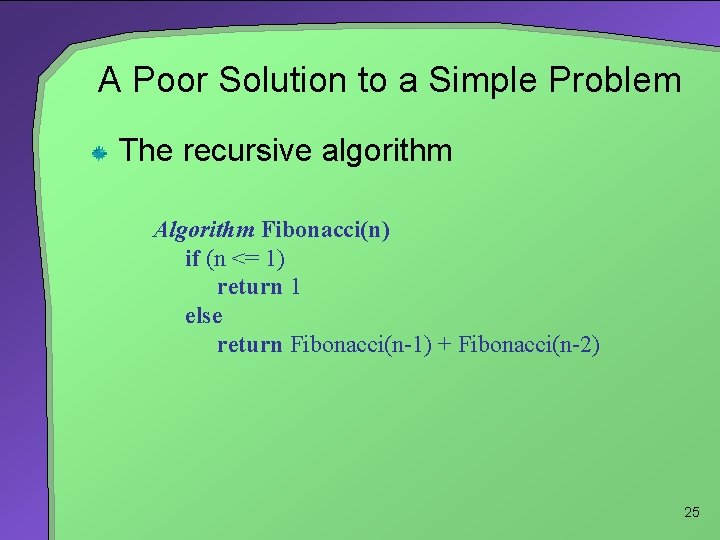A Poor Solution to a Simple Problem The recursive algorithm Algorithm Fibonacci(n) if (n <= 1) return 1 else return Fibonacci(n-1) + Fibonacci(n-2) 25A Poor Solution to a Simple Problem Time efficiency grows exponentially with n Iterative solution is O(n) Fig. 10 -10 The computation of the Fibonacci number F 6 (a) recursively; (b) iteratively 26Tail Recursion Occurs when the last action performed by a recursive method is a recursive call public static void count. Down(int integer) { System. out. println(integer); if (integer > 1) count. Down(integer - 1); } // end count. Down This performs a repetition that can be done more efficiently with iteration Conversion to iterative method is usually straightforward 27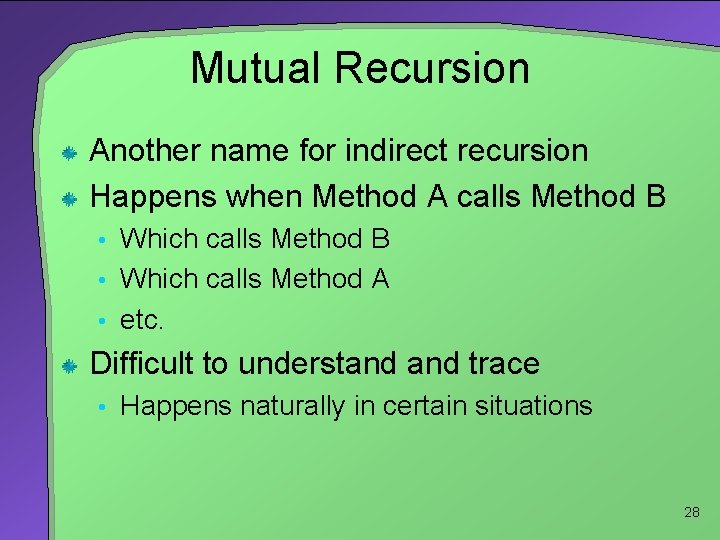Mutual Recursion Another name for indirect recursion Happens when Method A calls Method B • Which calls Method A • etc. Difficult to understand trace • Happens naturally in certain situations 28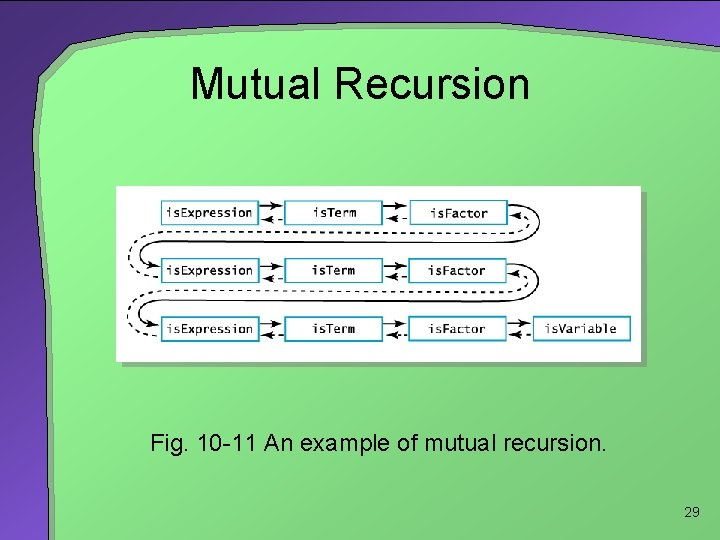Mutual Recursion Fig. 10 -11 An example of mutual recursion. 29